• 电机控制中，使用最多的就是PI控制器了，因为D参数通常使用不多。我打算以PMSM双环控制为例，对传统的PI控制进行分析。 下面是看到的一段PI控制器的介绍 按偏差的比例（P）、积分（I）和微分（D）进行控制的PID控制...
前言
电机控制中，使用最多的就是PI控制器了，因为D参数通常使用不多。我打算以PMSM双环控制为例，对传统的PI控制进行分析。
下面是看到的一段PI控制器的介绍

按偏差的比例（P）、积分（I）和微分（D）进行控制的PID控制器（亦称PID调节器）是应用最为广泛的一种自动控制器。它具有原理简单，易于实现，适用面广，控制参数相互独立，参数的选定比较简单等优点；而且在理论上可以证明，对于过程控制的典型对象──“一阶滞后+纯滞后”与“二阶滞后+纯滞后”的控制对象，PID控制器是一种最优控制。PID调节规律是连续系统动态品质校正的一种有效方法，它的参数整定方式简便，结构改变灵活（PI、PD、…）。

PI控制器具有一定的鲁棒性，因为它本身的定义上看，可以对误差校正，且校正本身不依赖于电机参数，只是PI控制器自身参数不合适的情况下，PI调节的动态性会不满意，最终稳态值可能也会有震荡。
PI控制器因为用的较多，参数设计方法很成熟。在使用FOC控制电机的情况下，电拖书里的直流电机双环参数设计方法可以被用于永磁同步电机的参数设计。当然，各家厂商对PI控制器的参数设计都提出过相应的设计方法，我个人是比较倾向于使用它们的设计方法，毕竟电拖书中要求PI控制器设计为I型和II型系统时，需要已知系统本身的延迟时间等参数，而这些参数比较难获得，厂商提供的电流环设计是按照一阶惯性环节设计的，还是比较简单的。
芯片厂商方案PI控制设计
ST的双环设计
电流环设计
设计时首先考虑进行电流环参数设计。当电机处于中低速运行时，可以忽略电流环输出的电压耦合项，电机的电流环PI参数配置，应当将PI调节器与电机构成一个闭环系统来看。
假设现在反电势电压为常数（因为通常相对于电流来说反电势电压变化缓慢），可将从电机电压到电机电流的小信号传输函数定义为：
$\frac{{I(s)}}{{V(s)}} = \frac{{\frac{1}{{{R_S}}}}}{{1 + \frac{{{L_S}}}{{{R_S}}}s}}$在这个系统中，按照零极消除的原则来配置PI参数，可以使得系统稳定。其中的AB为采样系数，这里可以认为都是1，即用真实值计算。根据ST等官方的参考手册，
使 $\frac{{{K_p}}}{{{K_I}}} = \frac{{{L_s}}}{{{R_s}}}$ ，则有可能实现零极消除；
在此情况下，闭环系统被还原成一阶系统；
可使用适当的KI值达到闭环系统要求的动态特性。这样设计完成后，电机的电流环控制系统如上，此时，系统等效于一个一阶低通滤波器。并且有
$\begin{array}{l} G(s) = \frac{1}{{\tau s + 1}}\\ = \frac{1}{{\frac{{{R_s}}}{{{K_I}}}s + 1}}\\ = \frac{1}{{\frac{{{L_s}}}{{{K_P}}}s + 1}} \end{array}$
那么，PI参数应该按照下面来配置，同时为了方便积分因子的计算，把采样频率SAMPLING_FREQ包含在KI表达式中（这一点非常重要，KI参数设计必须要考虑离散化）
$\begin{array}{l} {K_p} = {L_s}{\omega _c}\\ {K_I} = \frac{{{R_s}{\omega _c}}}{{{f_k}}} \end{array}$
${\omega _c}$为闭环带宽。比如使 ${\omega _c} = 1500{\rm{ }}rad/sec$，则电流控制系统的时间常数为0.66ms。
关于加入采样频率的具体推导是
${k_i}\int\limits_0^t {e(\tau )} d\tau = {k_i}{T_s}\sum\limits_{k = 1}^n {e(k{T_s}) = } {K_I}\sum\limits_{k = 1}^n {e(k{T_s})}$
式中采样周期就是采样频率的倒数。
总结下来，这一方法把电流环设计变为了单参数${\omega _c}$的调节，简化了电流环的设计。
速度环设计
转速环PI调节器的参数整定难度很大，关于电拖所用的参数整定方法，《现代永磁同步电机控制原理及MATLAB仿真》一书的评价是

采用自动控制理论中的典型II系统进行PI参数整定。虽然该方法具有一定的实际价值，但是Pl调节器参数整定的设计过程中涉及的中间变量较多。并且很多情况下是基于特定假设条件得到的近似结果。

所以我也不太推荐用II型系统法来进行速度环参数的设计。
ST早期的库说明中没有对速度环的参数设计介绍，但是在5.0的库中，我看到了参数设计。这个整定方法和有功阻尼法很像，所以我后面都是按照《现代永磁同步电机控制原理及MATLAB仿真》里面的有功阻尼法讲速度环参数设计
为了便于转速环PI调节器的参数整定，重写三相PMSM的电机运动方程为
$\begin{array}{l} J\frac{{d{\omega _m}}}{{dt}} = {T_e} - {T_L} - B{\omega _m}\\ {T_e} = \frac{3}{2}{p_n}{i_q}[{i_d}({L_d} - {L_q}) + {\psi _f}] \end{array}$
其中：${\omega _m}$为电机的机械角速度；J为转动惯量；B为阻尼系数；${T_L}$为负载转矩。
定义有功阻尼为
${i_q} = i_q^* - {B_a}{\omega _m}$
当采用id=0的控制策略，并假定电机在空载（TL=0）情况下启动时，由上面三式子可以得到
$\frac{{d{\omega _m}}}{{dt}} = \frac{{1.5{p_n}{\psi _f}}}{J}(i_q^* - {B_a}{\omega _m}) - \frac{B}{J}{\omega _m}$
将式中的极点配置到期望的闭环带宽，可以得到转速相对于q轴电流的传递函数为
${\omega _m}(s) = \frac{{1.5{p_n}{\psi _f}/J}}{{s + \beta }}i_q^*(s)$
计算有功阻尼的系数Ba:
${B_a} = \frac{{\beta J - B}}{{1.5{p_n}{\psi _f}}}$
若采用传统的PI调节器，则转速环控制器的表达式为
$i_q^* = ({K_{p\omega }} + \frac{{{K_{i\omega }}}}{s})(\omega _m^* - {\omega _m}) - {B_a}{\omega _m}$
因此，PI调节器的参数Kpw、Kiw可由下式整定
$\left\{ \begin{array}{l} {K_{p\omega }} = \frac{{\beta J}}{{1.5{p_n}{\psi _f}}}\\ {K_{i\omega }} = \beta {K_{p\omega }} \end{array} \right.$
其中：$\beta$是转速环期望的频带带宽。相对于采用典型II系统进行PI调节器参数整定的方法，这一参数整定方法简单，并且参数调整与系统的动态品质关系明确。
当然了，实际用的时候，I参数要除以速度环执行频率，现在的设计都是连续域下的。
ST的速度环计算公式
$s$域PI系数设定方法
$\begin{array}{l} {K_{P{\rm{ - }}ASR}} = J{\omega _{B - ASR}}\\ {K_{I{\rm{ - }}ASR}} = F{\omega _{B - ASR}} \end{array}$
式中：$J\left[ {{\rm{kg/}}{m^2}} \right]$为转动惯量，$F\left[ {Nm/\left[ {rad/s} \right]} \right]$为阻力系数，${\omega _{B - ASR}}\left[ {rad/s} \right]$为速度调节器通带宽度（即带宽），${K_{P{\rm{ - }}ASR}}$为速度调节器比例系数，${K_{I{\rm{ - }}ASR}}$为速度调节器积分系数。
当然，在使用ST的FOC库函数时，需要考虑WB（一个软件）的系数设定(具体需要了解ST库函数的标幺化)：
$\begin{array}{l} {K_{P{\rm{ - }}ASR - WB}} = \frac{{\frac{{{J_{WB}}}}{{1000000}}k\left( {2\pi {f_{B - ASR}}} \right)p}}{{10}}\\ {K_{I{\rm{ - }}ASR - WB}} = \frac{{{K_{P{\rm{ - }}ASR - WB}}}}{{{\tau _{mech}}}}\frac{{{T_{S - ASR - WB}}}}{{1000}} \end{array}$
式中：
$\begin{array}{l} {k_\tau } = 1.5\sqrt {\frac{2}{3}} \frac{{60}}{{1000}}\frac{1}{{2\pi }}\\ k = \frac{{65536{R_{shunt}}{G_{OPAMP}}}}{{3.3{k_\tau }}}\\ {\tau _{mech}} = \frac{{{J_{WB}}}}{{{F_{WB}}}}\\ {f_{B - ASR}} = \frac{{0.5}}{{{\tau _{mech}}}} \times 30 \end{array}$
TI参数设计方法
电流环设计TI的设计方法中，电流环的设计与ST的差不多，电流环 作为速度环PI调节器的内环。
有区别的是，PI的环路结构，注意TI里面的从上面的表达式可以看到，  ${K_P}$用于设定闭环系统响应的带宽， ${K_P}$越大，电流环路带宽越大。后面将分析如何选择合适的带宽。无论选择的带宽频率是多少，都可能会出现等于感抗的情况。
同时，$\frac{{{K_I}}}{{{K_P}}}$ 的配置是用于设定PI 控制器的零点。当控制自身传输函数中只有一个实极点的设备参数时（如电机中的电流）， $\frac{{{K_I}}}{{{K_P}}}$应将设为该实极点的值。这样可消除极点/零点并生成同样只有单个实极点的闭环响应。即非常稳定、不含谐振峰的响应。
目前，更常采用的是类似ST的PI控制器结构，故实际使用需要注意换算。
速度环设计
TI的速度环设计更像电拖的II型系统设计，分析得比ST的也全面多了。
基于前面中低速下的电流环PI参数，此时，PI 电流控制器作为内部环路的级联PI 速度环路。
速度环比电流环的设计更为复杂一些。另外，要正确设计速度环，还需要在电流环的基础上了解更多的系统参数。下图显示了构成级联速度控制环路的全部组件。所谓“级联”是指控制系统由具有一个或者更多内部环路的外部环路组成。${G_{current}}(s) = \frac{1}{{\frac{L}{{k_p^{series}}}s + 1}}$
用该传递函数代替电流环。
对于速度环，传递函数为
$\begin{array}{l} P{I_{speed}}(s) = \frac{{spdk_p^{series} \times spdk_i^{series}}}{s} + spdk_p^{series}\\ \begin{matrix} {}&{ = \frac{{spdk_p^{series} \times spdk_i^{series}(1 + \frac{s}{{spdk_i^{series}}})}}{s}} \end{matrix} \end{array}$
对PMSM，  ${i_q}$与电机转矩的传递函数为
$Mtr(s) = \frac{3}{2}p{\psi _f}$
那么，整个系统的开环传递函数为
$GH(s) = \left( {\frac{{spdk_p^{series} \times spdk_i^{series}(1 + \frac{s}{{spdk_i^{series}}})}}{s}} \right)\left( {\frac{1}{{\frac{L}{{k_p^{series}}}s + 1}}} \right)\left( {\frac{3}{2}p{\psi _f}} \right)\left( {\frac{1}{J}\frac{1}{s}} \right)$
简化式子
$\begin{array}{l} GH(s) = \frac{{K \times spdk_p^{series} \times spdk_i^{series}(1 + \frac{s}{{spdk_i^{series}}})}}{{{s^2}\left( {\frac{L}{{k_p^{series}}}s + 1} \right)}}\\ K = \frac{{3p{\psi _f}}}{{2J}} \end{array}$
这里存在三个极点
两个极点位于$s = 0$ 处，因此低频衰减率为每十倍频40Db.
另一个极点为 $s = - \frac{{K_p^{series}}}{L}$(电流控制器的极点)
还有一个零点 $s = - spdK_i^{series}$处。
为了保持稳定运行，$s = - \frac{{K_p^{series}}}{L}$ 极点的频率应高于$s = - spdK_i^{series}$零点频率。除此之外，还可以使用PI参数的无数种组合得到不同的系统响应，具体取决于是要更高的带宽还是要更高的稳定性。可以定义与系统稳定性成正比、与带宽成反比的单个参数，可使用该参数自动设置PI参数，从而产生所选带宽下的最大相位裕度。
当 $s = - \frac{{K_p^{series}}}{L}$的频率高于 $s = - spdK_i^{series}$时，并且单位增益频率介于上述两个频率之间，则得到的波特图应类似下图曲线形状之所以重要的原因是0dB 频率处的相移决定着系统稳定性。在极点频率与零点频率之间分离已确定的情况下，为使相位裕度最大化（相移：180°），0dB 频率的位置应恰好在对数刻度上极点频率与零点频率之间的中点位置。即，
$\begin{array}{l} {\omega _{0dB}} = \delta \times {\omega _{zero}}\\ {\omega _{pole}} = \delta \times {\omega _{0dB}}\\ {\omega _{pole}} = {\delta ^2} \times {\omega _{zero}} \end{array}$
那么，$spdK_i^{series} = \frac{{K_p^{series}}}{{{\delta ^2}L}}$
其中， $\delta$为阻尼因子。$\delta$值越大，零点角频率与电流环路极点频率之间的分离程度越大。分离程度越大，两个频率之间所能够达到的相位裕度峰值越大。这种稳定性的提升建立在牺牲速度环路带宽的基础上。$\delta= 1$ 时，零点角频率与电流环路极点频率相等，这样能够消除极点/零点并且系统将变得不稳定。由于相位裕度> 0，因此理论上只要$\delta>1$  时，系统都是稳定的。但是，$\delta$ 的值接近1 会导致性能上表现出严重的欠阻尼。
速度环路的开环传输函数在频率等于零点拐点频率与δ 的乘积时为单位增益
$\begin{array}{l} GH(s) = \frac{{K \times spdk_p^{series} \times spdk_i^{series}(1 + \frac{s}{{spdk_i^{series}}})}}{{{s^2}\left( {\frac{L}{{k_p^{series}}}s + 1} \right)}}\\ K = \frac{{3p{\psi _f}}}{{2J}} \end{array}$
${\left| {\frac{{K \times spdk_p^{series} \times spdk_i^{series}(1 + \frac{s}{{spdk_i^{series}}})}}{{{s^2}\left( {\frac{s}{{{\delta ^2} \times spdk_i^{series}}} + 1} \right)}}} \right|_{s = j \times \delta \times spdK_i^{series}}} = 1$
对上面式子 “s”进行指定替换和整理后，得到：
$\frac{{\delta \times K \times spdK_p^{series}}}{{{\delta ^2}(\frac{{K_p^{series}}}{{{\delta ^2} \times L}})}} = 1$
最终，可得
$spdK_p^{series} = \frac{{K_p^{series}}}{{L \times \delta \times K}} = \frac{{\delta \times spdK_i^{series}}}{K}$
据此，可以得到最后的速度环PI参数。
这种方法不用根据经验调整四个看似与系统性能相关性很小的PI 系数，而是只需要定义两个有意义的系统参数：电流控制器带宽和速度环路阻尼系数。同时，需要结合电机的模型获得电机的几个参数。
阻尼因子是代表系统稳定性与系统带宽之间权衡的一个数字。现在，已经对双环控制器的设计完成了简化，即四个PI 系数简化为两个“系统”参数。其中一个参数是电流控制器带宽。另一个是阻尼因子(δ)。这里的参数调节仅针对只考虑仅含转矩和惯性组件（即，无扭转谐振或粘滞阻尼）的负载。速度控制器开环幅度和相位响应是δ 的函数
由于电流带宽值的作用只是为频率轴提供参考点，因此其数值并不重要。但无论电流带宽是多少，曲线形状都不会改变。阻尼因子在1.5 至70 范围内扫描，分为8 个离散段，以显示如何对系统响应产生影响。值为1.0 时所对应的条件是：开环增益截距为0dB 且刚好在电流控制器带宽的频率处。这会导致在该频率处极点/零点消除且相位裕度为零。很明显，相位裕度为零对系统来说并不是件好事情。
阻尼因子方程的一个目标是在给定带宽条件下获得最大稳定性。对于上图中的某个频率，当开环相位曲线中的相位裕度峰值达到自身最大值时，开环增益曲线恰好通过0db。随着稳定性因子增加，最终会在信号相移接近-90 度时达到下降点。但是，增益裕度会继续增加，其结果是大幅降低系统响应速度。
根据TI的手册建议，得到以下计算电流环带宽的公式
$B{W_c} = \frac{{K_p^{series}}}{L} = B{W_S}(\delta + 2.16 \times {e^{\frac{\delta }{{2.8}}}} - 1.86)$
$B{W_s}$是速度控制器带宽,单位是rad/s。实际控制系统中，还存在速度滤波的环节。它会影响传递函数。同时，速度PI 控制器需要考虑的参数：电流限制、钳位和惯性。这些都会对PI参数的配置产生影响。
此外，矢量控制中，两个电流环相互之间存在的耦合影响，对此的解耦方案有前馈解耦等。$\begin{array}{l} {i_d}(R + p{L_s}) = {U_d} + \omega {L_s}{i_q}\\ {i_q}(R + p{L_s}) = {U_q} - \omega ({L_s}{i_q} + {\psi _f}) \end{array}$
注：国际标准单位下同时，采样和数据处理格式对PI调节器也有影响。在上图所示的数字控制系统中，系统在PWM 周期开始时通过ADC 对电机信号进行采样并执行相应的控制计算，然后将得到的控制电压存入双缓冲PWM 寄存器。上述值在PWM 模块中保持未使用状态，直到下一个PWM 周期开始时才会将其作为PWM 输出的时钟信号。从系统建模的角度看，这就相当于采样频率等于PWM 更新频率时的采样和保持功能。采样和保持功能的固定时间延迟表现为滞后相位角，频率越高时滞后情况越严重。
下图显示了采样和保持功能的标准化频率曲线图，假设采样频率为1。相位曲线是最重要的图形，因为它显示了采样和保持功能的相位延迟频率能够达到远低于采样频率的水平。例如，即使在采样频率的十分之一处，采样和保持(S&H) 功能仍然会产生-18 度的相移。针对低载波比下的数字控制系统，尽管不存在完美比率，但通常倾向于使用经验法则，即采样频率应至少为电流控制器带宽(BWc) 的10 倍。这样可确保S&H 相位延迟的影响仅从电流控制器的相位裕度中减去18 度，从而得到非常稳定的72 度相位裕度。当然，如有需要还可设置更高的采样频率，但这通常需要使用具有更高MIPS 的更加昂贵的处理器。
假如系统响应迟缓且电机输出似乎未达到额定转矩，则可能是系统中粘滞阻尼过大。
总结
目前有许多新的控制算法，像滑模控制、模型预测控制等，这些算法可能会有更好的控制效果，但是PI控制的参数设计还是有必要学习的，毕竟PI控制器在普通性能的单片机上都能跑，而且它的经典性也是毋庸置疑的。


展开全文pid
• ## 数字PI控制器

千次阅读 2015-04-18 20:44:59
数字PI控制器是控制系统中常用的简单有效的控制算法， 用C语言实现该算法，使用与各类嵌入式控制系统中
最近项目需要用到PI控制器，于是参考网上的一些资料，尝试着写了一下，将它记录下来以备后用。

PID公式：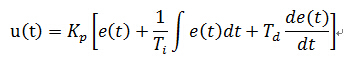Ti，Td分别为积分，微分常数，只取PI部分：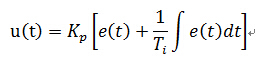根据采样周期T离散化，t=nT,用n表示，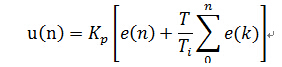可得增量式：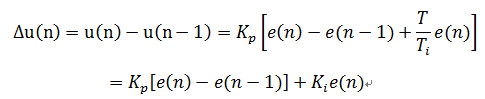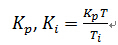分别为比例，积分系数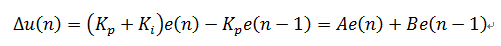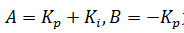为计算系数

最终输出为：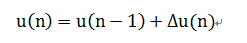写代码时，定义一个结构体来存放各个参数，
程序设计流程为：

本次采样输入→计算本次误差→计算输出增量→更新数据为下次做准备
附源码：
/************声明和定义，通常放在头文件中********/
typedef struct
{
int SetValue;        //设置值
int An;			//当前误差e(n)的系数，=kp+ki
int Bn_1;			//前一项误差e(n-1)的系数，= -kp
int Limit;			//限制最大最小值
int Errorn_1;		//e(n-1)
int DeltaUn;		//输出增量△u(n);
int Un_output;	//输出值 u(n)
}PI_Typedef;

void PI_StructInit(PI_Typedef *PI_Struct,int Kp,int Ki,int limit);
void PI_Controller(PI_Typedef *PI_Struct,int NewInput,int *Output);
/*****************************************************************/

void PI_StructInit(PI_Typedef *PI_Struct,int Kp,int Ki,int limit)
{
PI_Struct->SetValue = 0;
PI_Struct->An = Kp+Ki;
PI_Struct->Bn_1 = -Kp;
PI_Struct->Limit = limit;
PI_Struct->DeltaUn = 0;
PI_Struct->Errorn_1 = 0;
}

void PI_Controller(PI_Typedef *PI_Struct,int NewInput,int *Output)
{
int Errorn =0;

Errorn = PI_Struct->SetValue - NewInput;//计算e(n)

PI_Struct->DeltaUn = (PI_Struct->An)*Errorn+(PI_Struct->Bn_1)*(PI_Struct->Errorn_1);//计算增量
PI_Struct->Errorn_1 = Errorn;//保存误差e(n)作为下一次计算的e(n-1)
//限制增量的范围
if(PI_Struct->DeltaUn > PI_Struct->Limit)
{PI_Struct->DeltaUn = PI_Struct->Limit;}
else if(PI_Struct->DeltaUn < -PI_Struct->Limit)
{PI_Struct->DeltaUn = -PI_Struct->Limit;}

PI_Struct->Un_output += PI_Struct->DeltaUn; //计算输出u(n)=u(n-1)+△u(n),并保存作下一次运算

*Output = PI_Struct->Un_output;
}

wind

2015,04,18


展开全文PI算法 c语言
基于MATLAB的模糊pi控制器的设计
% 模糊规则隶属函数的建立
a=newfis(‘fuzzypid’);
% 添加第一个输入变量e
% 添加第二个输入变量ec
% 添加第一个输出变量kp
% 添加第二个输出变量ki
% 添加模糊规则
rulelist=[
1 1 1 2 1 1;
1 2 1 2 1 1;
1 3 1 2 1 1;
2 1 1 3 1 1;
2 2 3 3 1 1;
2 3 3 3 1 1;
3 1 3 2 1 1;
3 2 3 2 1 1;
3 3 3 2 1 1
];
% 将模糊规则加入到a中
% 解模糊
a=setfis(a,‘defuzzmethod’,‘centroid’);
% 保存到系统磁盘中
writefis(a,‘fuzzypid’);
% 读取模糊推理系统
% 作图
figure(1);
plotmf(a,‘input’,1);
figure(2);
plotmf(a,‘input’,2);
figure(3);
plotmf(a,‘output’,1);
figure(4);
plotmf(a,‘output’,2);
figure(5);
plotfis(a);
showrule(a)
ruleview fuzzypid;
% 模糊pid主控程序
% 加载模糊规则
% 建立控制对象的模型
ts=0.001;                      %设定步长
sys=tf(133,[1,25,0]);          %将连续型传递函数通过z变换为离散型
dsys=c2d(sys,ts,‘z’);          %离散化
[num,den]=tfdata(dsys,‘v’);    %获得离散建模的分子分母
% 设定系统相关参数的初值
u_1=0;u_2=0;
y_1=0;y_2=0;
e_1=0;ec_1=0;ei=0;
kp0=0;ki0=0;
for k=1:1:1000
time(k)=k*ts;    %设定步数
rin(k)=1;
k_pid=evalfis([e_1,ec_1],a);  %离散化后提取分子、分母
kp(k)=kp0+k_pid(1);
ki(k)=ki0+k_pid(2);
u(k)=kp(k)*e_1+ki(k)*ei;

yk=-den(2)*y_1-den(3)*y_2+num(2)*u_1+num(3)*u_2;
e(k)=rin(k)-y(k);

u_2=u_1;
u_1=u(k);
y_2=y_1;
y_1=y(k);

ei=ei+e(k)*ts;
ec(k)=(e(k)-e_1)/ts;

e_1=e(k);
ec_1=ec(k);

end
figure(1);
plot(time,rin,‘r’,time,y,‘b’,‘linewidth’,1);
xlabel(‘time(s)’);
ylabel(‘yd,y’);
legend(‘ideal position’,‘position tracking’);
figure(2);
plot(time,kp,‘r’,‘linewidth’,1);
xlabel(‘time(s)’);
ylabel(‘kp’);
figure(3);
plot(time,ki,‘k’,‘linewidth’,1);
xlabel(‘time(s)’);
ylabel(‘ki’);
figure(4);
plot(time,u,‘r’,‘linewidth’,1);
xlabel(‘time(s)’);
ylabel(‘control input’);
结果显示：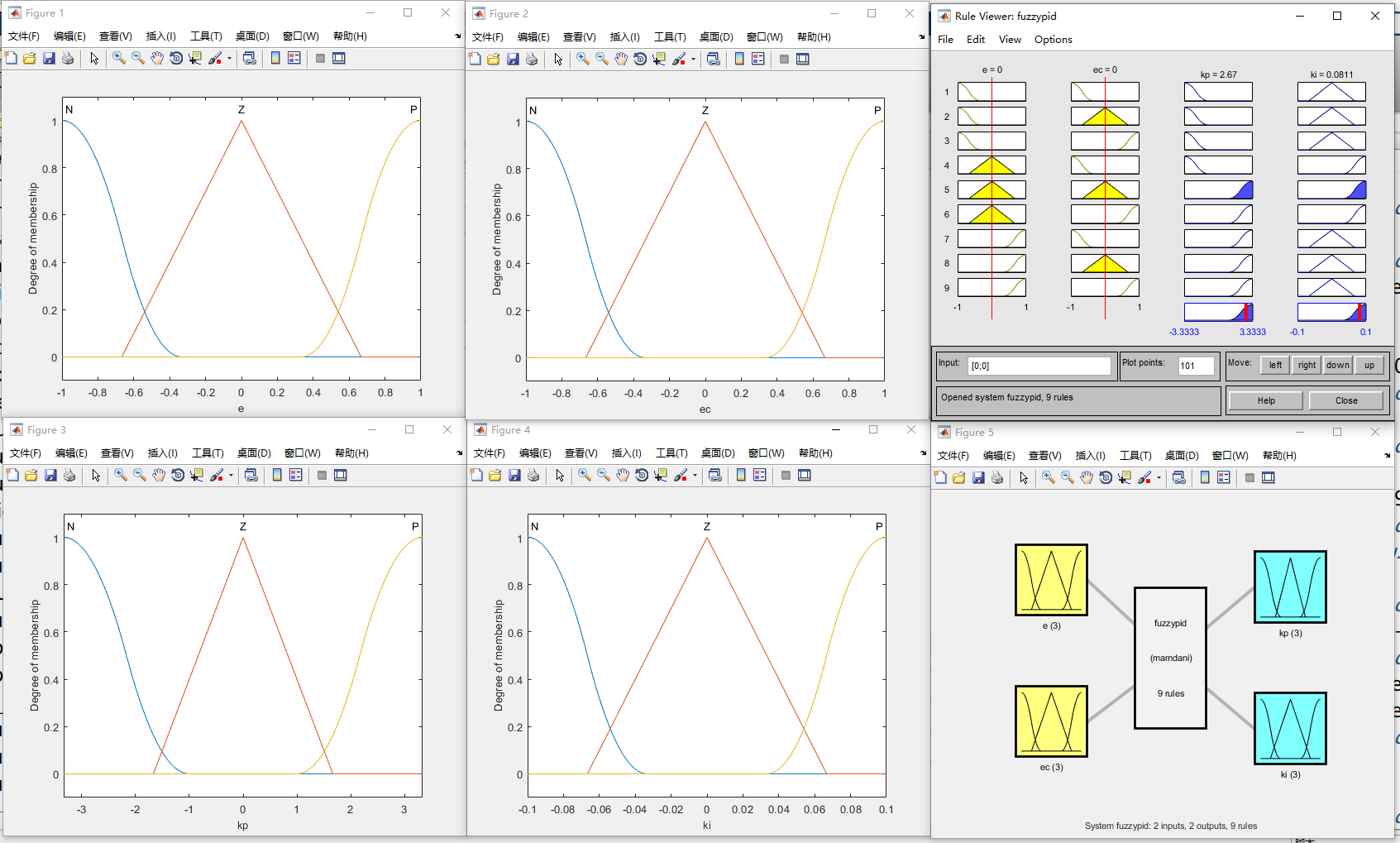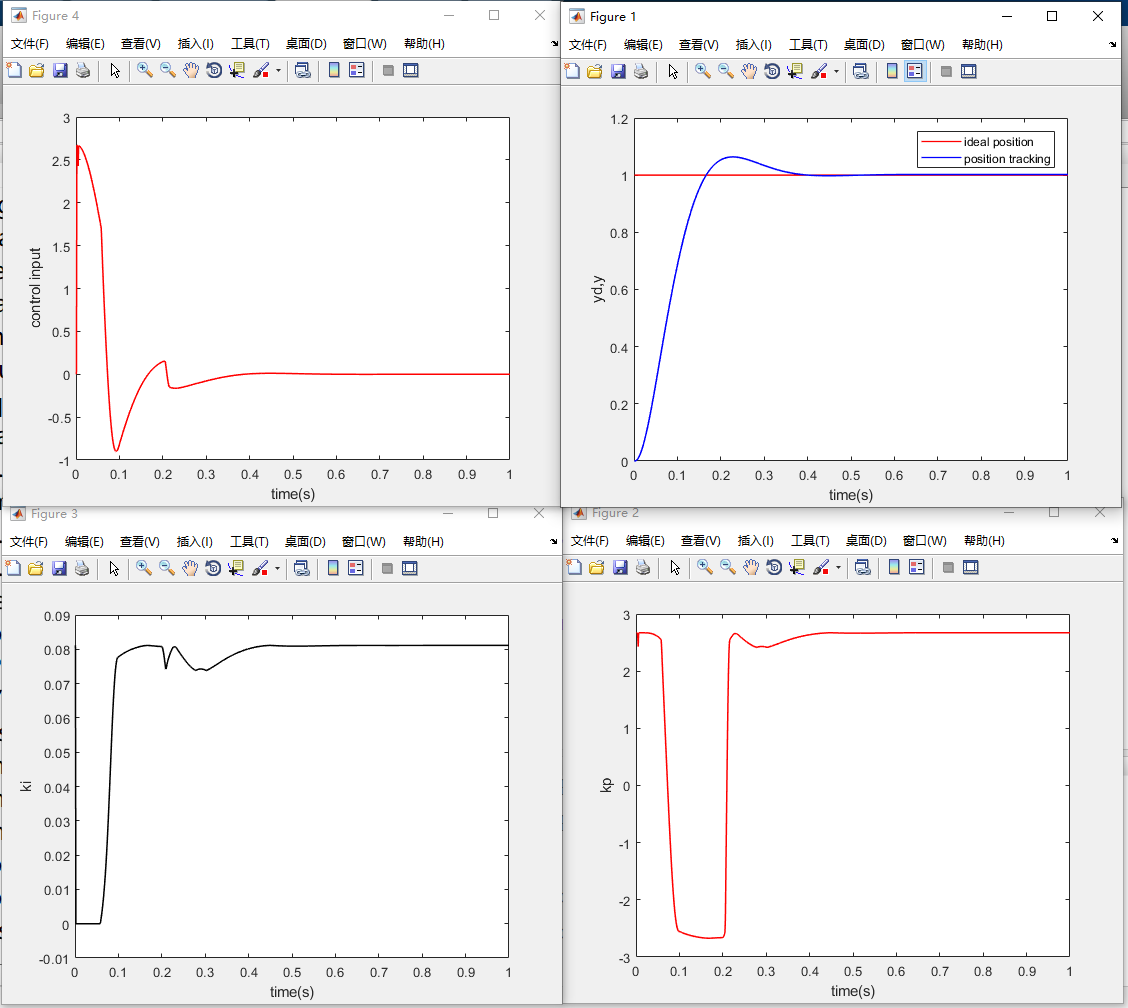展开全文matlab pid
• 最近在做永磁同步电机控制器的毕业设计，要求双闭环系统，PI控制，使用SVPWM算法，有没有详细程序与有关详解。c语言
• Waveshare给您介绍一款树莓派家族的新成员，Raspberry Pi Pico 是一款低成本，高性能的微控制器开发板，具有灵活数字接口。 硬件上，采用Raspberry Pi 官方自主研发的 RP2040 微控制器芯片，搭载了ARM Cortex M0 + ...
Waveshare给您介绍一款树莓派家族的新成员，Raspberry Pi Pico 是一款低成本，高性能的微控制器开发板，具有灵活数字接口。

硬件上，采用Raspberry Pi 官方自主研发的 RP2040 微控制器芯片，搭载了ARM Cortex M0 + 双核处理器，高达133MHz的运行频率，内置了264KB的SRAM和2MB的内存，还板载有多达26个多功能的GPIO引脚。尺寸小巧：21*51MM，PCB设计成邮票型焊盘，方便用户集成到自己的产品当中。

软件上，可选择树莓派提供的C/C++ SDK，或者使用MicroPython进行开发，且配套有完善的开发资料教程，可方便快速入门开发，并嵌入应用到产品中。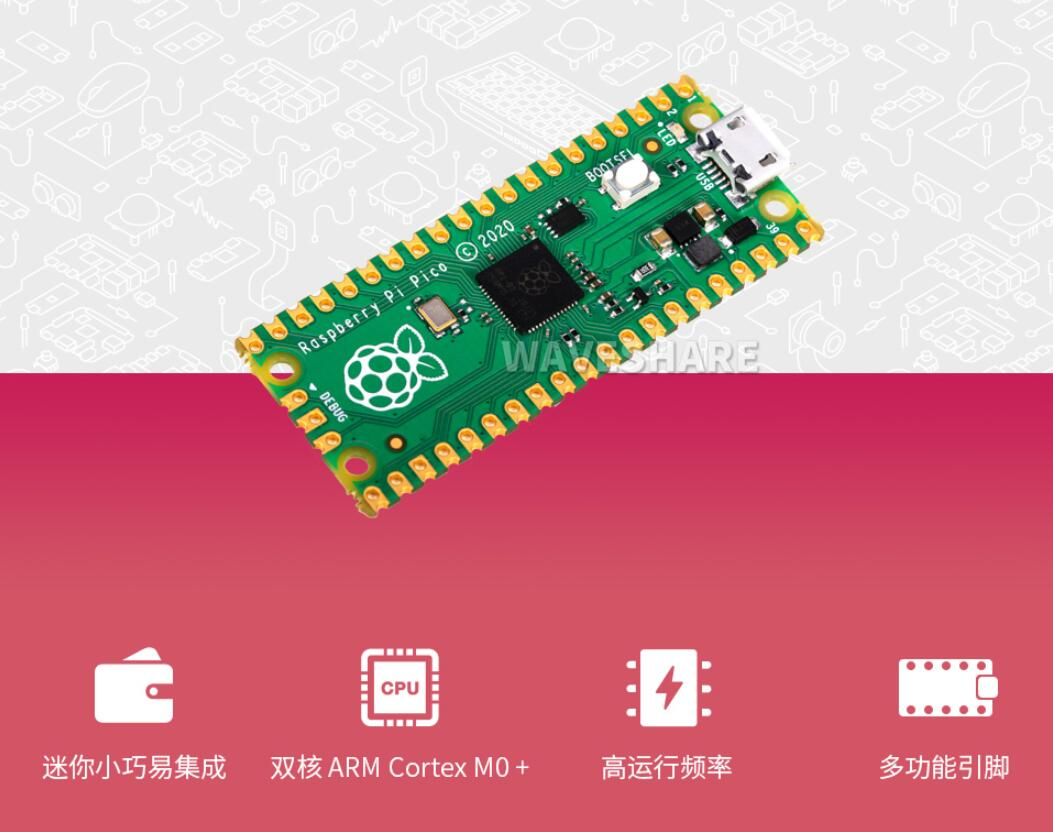主要功能特性有：

采用了 Raspberry Pi 官方自主设计的RP2040 微控制器芯片
搭载了双核 ARM Cortex M0 + 处理器，运行频率高达 133 MHz 灵活时钟
内置了 264KB 的 SRAM 和 2MB 的片上Flash
邮票孔设计，可直接焊接集成到用户自主设计的底板上
USB1.1 主机和设备支持
支持低功耗睡眠和休眠模式
可通过 USB 识别为大容量存储器进行拖放式下载程序
多达 26 个多功能的 GPIO 引脚
2 个 SPI，2 个 I2C，2 个 UART，3 个 12 位 ADC，16 个可控 PWM 通道
精确的片上时钟和定时器
温度传感器
片上加速浮点库
8 个可编程 I/O (PIO) 状态机，用于自定义外设支持
树莓派PICO拥有26 个多功能 GPIO 引脚

支持配置引脚功能，可方便灵活地开发和集成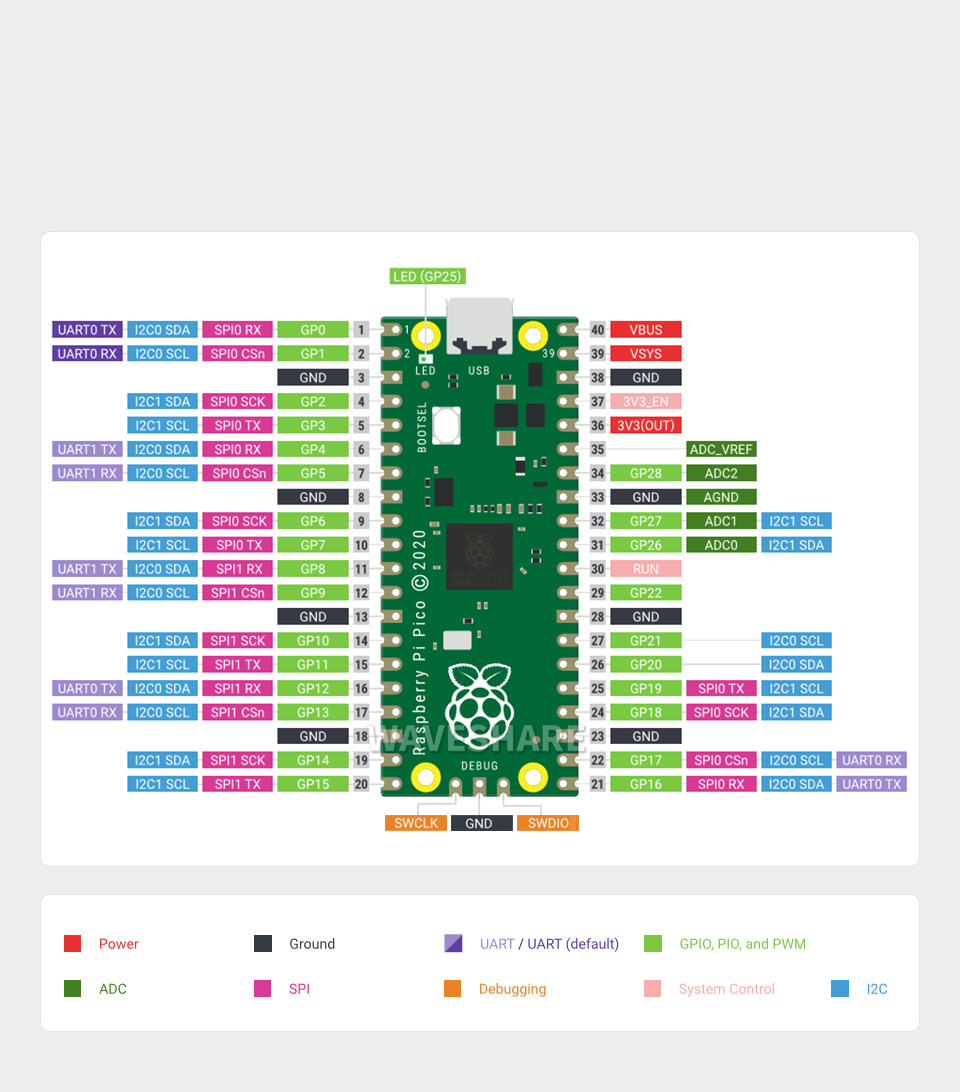树莓派PICO的包装采用的是编带的，方便客户批量生产。树莓派这次挺看重这款Raspberry Pi Pico 的，这款型号并没有做预售，直接现货发售，微雪电子本次也是拿到了第一批现货。

感兴趣的小伙伴们可以入手了，手快有手慢就要等了。

评论中有链接可以登陆查看。

另外使用教程已更新，有兴趣的板友们可以去看看→https://blog.csdn.net/weixuedianzi/article/details/113366672


展开全文单片机 树莓派
• ​ 下图是在12V供电，1.7bar的压力条件下，比例阀的流量与控制电流之间的关系曲线，可以发现比例阀的流量控制具有滞环的特点。 ​ 下图则是比例阀的流量与入口压力之间的关系曲线， 从图中可以看出流量随着压力的...c语言 pid matlab
• 感应（异步）电机磁场定向控制电流环PI控制参数设计 目录电机控制系列文章前言一、速度环开环传递函数二、速度环开环传递函数零极点配置三、速度环闭环性能四、速度滤波器的影响总结 前言 大家在做感应（异步）...matlab simulink
• 基于MATLAB的单相PWM逆变仿真 带PI控制 带数学建模
• 利用matlab搭建了PI双环控制的三相逆变的仿真
• 主要包括三个部分：转速环PI调节，电流环PI调节，SVPWM算法等。 主要参数计算 3.1 转速环ADRC理论分析 请参考赠送的文献或者硕士论文。谢谢。 3.2 电流环PI参数理论分析 3.3 电流环PI参数...永磁同步电机 SVPWM
• 本篇文章首发于公众号：无人机系统技术。更多无人机技术相关文章请关注此公众号。...接下来的几篇内容将以多旋翼飞行器为例，介绍PID控制方法在控制器设计中的应用，还将以px4开源软件为例说明控制器设计的具体实...无人机 PID 入门
• 1.什么是滞后补偿以及和PI控制的联系 2.PI控制对系统的影响 3.如何设计一个运动控制系统 4.抗积分饱和的方法 5.一个实例 1.什么是超前补偿以及和PI控制的联系 上一篇文章提到了超前补偿，超前这个词的含义...算法
• 一般伺服驱动都具备位置、速度、和电流控制，框图如下图所示： 伺服驱动的电流环通常采用矢量控制方法，在很多电机控制书籍中都有很详细的介绍，本文结合具体实例，简单介绍其设计步骤。 一、电机状态方程 以...
• 目录系统模型及参数控制性能目标PID概述比例控制PI 控制器PID 控制器 系统模型及参数 前面教程已经得出了定速巡航系统的传递函数模型： P(s)=V(s)U(s)=1ms+b[m/sN] P(s) = \frac{V(s)}{U(s)} = \frac{1}{ms+b} \...MATLAB PID
• 为了使得开关电源有更好的稳态性能和动态性能，需要针对...本文简述了补偿的过程，以Buck开关电源为例，对未补偿系统进行分析，并设计补偿网络，最后对补偿后系统进行分析。经过分析，补偿后的系统性能优于补偿前系统。开关电源
• 3 PI控制器参数整定 3.1从PMSM电机的数学模型出发。 dq 轴 电压方程： dq 轴 轴磁链方程： dq 轴 转矩方程： dq 轴 运动方程： 分析上述方程，如果我们能够控制 id=0 那么电压...永磁同步电机
• [控制理论] （1） 二阶系统的滑模控制器设计[控制理论] （1） 二阶系统的滑模控制器设计1 前言 [控制理论] （1） 二阶系统的滑模控制器设计 本博客需要一些现代控制理论中Lyapunov稳定性的一些理论知识。 前文对...
• 这一节我们将对上一节的Buck电路进行闭环控制，控制方法采用最经典的PI控制器。 目标电压为15V。 电路的参数如下： PI的传递函数如下： 通过频率设计设计带宽为278rad/s，相位裕量为60°。则可以...
• 很少有小型的工业操作需要复杂的操作环境、高的I/O容量和复杂的功能需求，而这些要求是可编程逻辑控制器(PLCs)的初衷。虽然缩小版的PLCs是可用的，但设计师现在也可以选择从一系列低成本的开源硬件和软件解决方案中...
• 模糊PI控制器由常规PI控制和模糊推理两部分组成，模糊推理部分的输入是偏差E和偏差变化率EC，输出量是、。PI参数模糊自整定就是找出PI参数和，之间的模糊关系，在运行中不断检测和，根据模糊控制原理来对三个参数...PID 模糊PID
• 一、基本概念 对于图1所示系统，u为控制输入，y为测量输出，z为被调输出，w为干扰输入，由输入u，w到输出y，z的传递函数G成为...二、H无穷控制器设计方法 1. 基于Riccati方程的H∞控制设计 2.基于LMI的H∞控制 ...
• 整理 | 郑丽媛来源 | CSDN（ID：CSDNnews）昨天，树莓派搞了个大动作：推出了首款微控制器开发板Raspberry Pi Pico！该开发板基于树莓派开发的全新芯片——R...微软 cstring kernel arduino
• 倒立摆系统是一种典型控制对象，它为各种控制器设计方法提供了一个很好的实验和应用平台。文章首先依据一阶倒立摆系统的动力学方程对其进行了建模，得出了系统的传递函数和状态空间，其次利用PID控制、极点配置、...倒立摆
• ——《Fundamentals of Power Electrics》译文系列（之）控制器设计 1 概述 2 负反馈对网络传递函数的影响 2.1 反馈环节的特点：减小“扰动-输出”传递函数 2.2 反馈环节的特点：降低“给定-输出”传递函数对前向...
• 4.1 基于状态反馈的控制器设计 4.2 基于输出反馈的控制器设计 4.3. 基于观测器的控制器 1、观测器提出的背景 控制理论中的大多数概念是基于传感器来测量被控量的，事实上在讲授控制理论时，通常假定可采用近乎...
• 滑模控制器的理论设计与仿真实现 滑模控制器的设计与仿真实现，可以具体参考我之前写的博客...假定电流环均都是PI控制器，人为已经是整定至最佳的状态，从而来考量改进的效果与创新点。 图1 转速环为滑模控制的矢量控
• 永磁同步电机控制之反馈解耦及复矢量解耦一文对常用的反馈解耦、复矢量解耦进行了详细讲解，但现在的电机控制器基本使用数字控制器如TI的DSP、英飞凌的ARM等。故在离散域直接设计电流调节器可以获得更好的性能。 一...算法 c语言 经验分享...